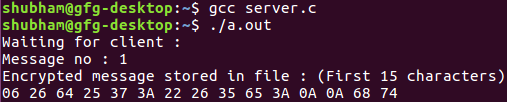# Message encryption and decryption using UDP server

The process of message encryption and decryption during client-server communication using UDP server is as follows:

• The client requests the server with a file name.
• The corresponding file is opened by the server and sends the file using datagram socket.
• The sender sends the encrypted text (Xoring) with a fixed length key.
• The receiver decrypts the file using the same key (private key).

Prerequisite : Socket Programming

Examples:

```Input :  file.txt
Output : ClientSide : Received from server:
00 2C 28 28 2A 6E 3B 24 37 64 65 79 0A 00 00
Decrypted message: Hello People
ServerSide : Encrypted message stored in file:
00 2C 28 28 2A 6E 3B 24 37 64 65 79 0A 00
```

Client Side Program:

 `// Cient side code ` `#include ` `#include ` `#include ` `#include ` ` `  `// Driver code ` `int` `main() ` `{ ` `    ``int` `clientSocket, portNum, nBytes; ` `    ``char` `file_buffer, path, buffer; ` ` `  `    ``// This key array stores the hidden key ` `    ``char` `const` `key = ``"HIDDENKEY"``; ` `    ``struct` `sockaddr_in serverAddr; ` `    ``socklen_t addr_size; ` `    ``int` `i; ` `    ``clientSocket = socket(PF_INET, SOCK_DGRAM, 0); ` ` `  `    ``serverAddr.sin_family = AF_INET; ` `    ``serverAddr.sin_port = htons(5004); ` `    ``serverAddr.sin_addr.s_addr = inet_addr(``"127.0.0.1"``); ` `    ``memset``(serverAddr.sin_zero, ``'\0'``, ``sizeof` `serverAddr.sin_zero); ` ` `  `    ``addr_size = ``sizeof` `serverAddr; ` ` `  `    ``while` `(1) { ` `        ``printf``(``"Specify file name: \n"``); ` `        ``gets``(path); ` ` `  `        ``// printf("%s\n", path); ` `        ``FILE``* fp; ` `        ``fp = ``fopen``(path, ``"r"``); ` `        ``if` `(fp == NULL) { ` `            ``printf``(``"file does not exist\n"``); ` `        ``} ` ` `  `        ``fseek``(fp, 0, SEEK_END); ` `        ``size_t` `file_size = ``ftell``(fp); ` `        ``fseek``(fp, 0, SEEK_SET); ` ` `  `        ``if` `(``fread``(file_buffer, file_size, 1, fp) <= 0) { ` `            ``printf``(``"unable to copy file into buffer\n"``); ` `            ``exit``(1); ` `        ``} ` ` `  `        ``if` `(sendto(clientSocket, file_buffer, 3000, 0, (``struct` `sockaddr*)&serverAddr,  ` `                                                                    ``addr_size) < 0) { ` `            ``printf``(``"error in sending the file\n"``); ` `            ``exit``(1); ` `        ``} ` ` `  `        ``bzero(file_buffer, ``sizeof``(file_buffer)); ` ` `  `        ``nBytes = recvfrom(clientSocket, buffer, 1024, 0, NULL, NULL); ` ` `  `        ``printf``(``"Received from server: \n"``); ` ` `  `        ``// printing some of the character to have a feel of encryption ` `        ``for` `(i = 0; i < 15; ++i) ` `            ``printf``(``"%02X "``, buffer[i]); ` `        ``printf``(``"\n"``); ` ` `  `        ``char` `x; ` `        ``for` `(i = 0; i < nBytes - 1; ++i) ` `            ``x[i] = (``char``)(buffer[i] ^ key[i]); ` ` `  `        ``// printing some of the character to have a feel of decryption ` `        ``printf``(``"Decrypted message: (First 15 characters)\n"``); ` `        ``for` `(i = 0; i < 11; ++i) ` `            ``printf``(``"%c "``, x[i]); ` ` `  `        ``printf``(``"\n"``); ` `    ``} ` ` `  `    ``return` `0; ` `} `

Server Side Program:

 `// C server code ` `#include ` `#include ` `#include ` `#include ` `#include ` `#include ` ` `  `// Driver code ` `int` `main() ` `{ ` `    ``int` `udpSocket, nBytes; ` `    ``char` `buffer, xor; ` `    ``char` `const` `key = ``"HIDDENKEY"``; ` `    ``struct` `sockaddr_in serverAddr, clientAddr; ` `    ``struct` `sockaddr_storage serverStorage; ` `    ``socklen_t addr_size, client_addr_size; ` `    ``int` `i; ` ` `  `    ``udpSocket = socket(PF_INET, SOCK_DGRAM, 0); ` ` `  `    ``serverAddr.sin_family = AF_INET; ` `    ``serverAddr.sin_port = htons(5004); ` `    ``serverAddr.sin_addr.s_addr = inet_addr(``"127.0.0.1"``); ` `    ``memset``(serverAddr.sin_zero, ``'\0'``, ``sizeof` `serverAddr.sin_zero); ` ` `  `    ``bind(udpSocket, (``struct` `sockaddr*)&serverAddr, ``sizeof``(serverAddr)); ` ` `  `    ``addr_size = ``sizeof` `serverStorage; ` `    ``puts``(``"Waiting for client :"``); ` `    ``int` `count = 0; ` ` `  `    ``while` `(1) { ` `        ``nBytes = recvfrom(udpSocket, buffer, 3000, 0, (``struct` `sockaddr*)&serverStorage,  ` `                                                                            ``&addr_size); ` `        ``printf``(``"Message no : %d\n"``, ++count); ` ` `  `        ``for` `(i = 0; i < nBytes - 1; i++) { ` `            ``if` `(buffer[i] != ``'\n'``) ` `                ``xor[i] = (``char``)(buffer[i] ^ key[i]); ` `            ``else` `                ``xor[i] = buffer[i]; ` `        ``} ` ` `  `        ``printf``(``"Encrypted message stored in file : (First 15 characters)\n"``); ` ` `  `        ``// printing some of the character to have a feel of encryption ` `        ``for` `(i = 0; i < 15; ++i) ` `            ``printf``(``"%02X "``, xor[i]); ` `        ``printf``(``"\n"``); ` ` `  `        ``FILE``* fp; ` `        ``fp = ``fopen``(``"temp.txt"``, ``"w+"``); ` ` `  `        ``for` `(i = 0; i < nBytes - 1; i++) { ` `            ``if` `(xor[i] != ``'\n'``) ` `                ``fprintf``(fp, ``"%X"``, xor[i]); ` `            ``else` `                ``fprintf``(fp, ``"%c"``, xor[i]); ` `        ``} ` ` `  `        ``fclose``(fp); ` `        ``sendto(udpSocket, xor, nBytes, 0, (``struct` `sockaddr*)&serverStorage,  ` `                                                                 ``addr_size); ` `    ``} ` ` `  `    ``return` `0; ` `} `

Output :Attention reader! Don’t stop learning now. Get hold of all the important DSA concepts with the DSA Self Paced Course at a student-friendly price and become industry ready.

My Personal Notes arrow_drop_upCheck out this Author's contributed articles.

If you like GeeksforGeeks and would like to contribute, you can also write an article using contribute.geeksforgeeks.org or mail your article to contribute@geeksforgeeks.org. See your article appearing on the GeeksforGeeks main page and help other Geeks.

Please Improve this article if you find anything incorrect by clicking on the "Improve Article" button below.

Article Tags :
Practice Tags :

Be the First to upvote.

Please write to us at contribute@geeksforgeeks.org to report any issue with the above content.